# Problem 10: 10 points Assume that a sample {X;:15; <4} of size 4 is drawn from...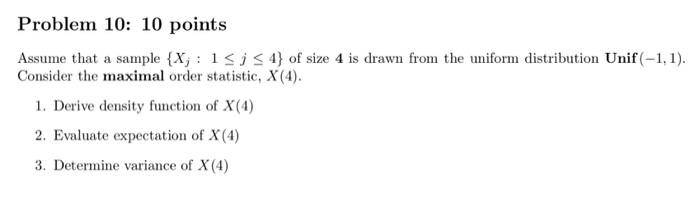Problem 10: 10 points Assume that a sample {X;:15; <4} of size 4 is drawn from the uniform distribution Unif(-1,1). Consider the maximal order statistic, X(4). 1. Derive density function of X(4) 2. Evaluate expectation of X(4) 3. Determine variance of X(4)

TOPIC:Distribution of the maximal order statistic.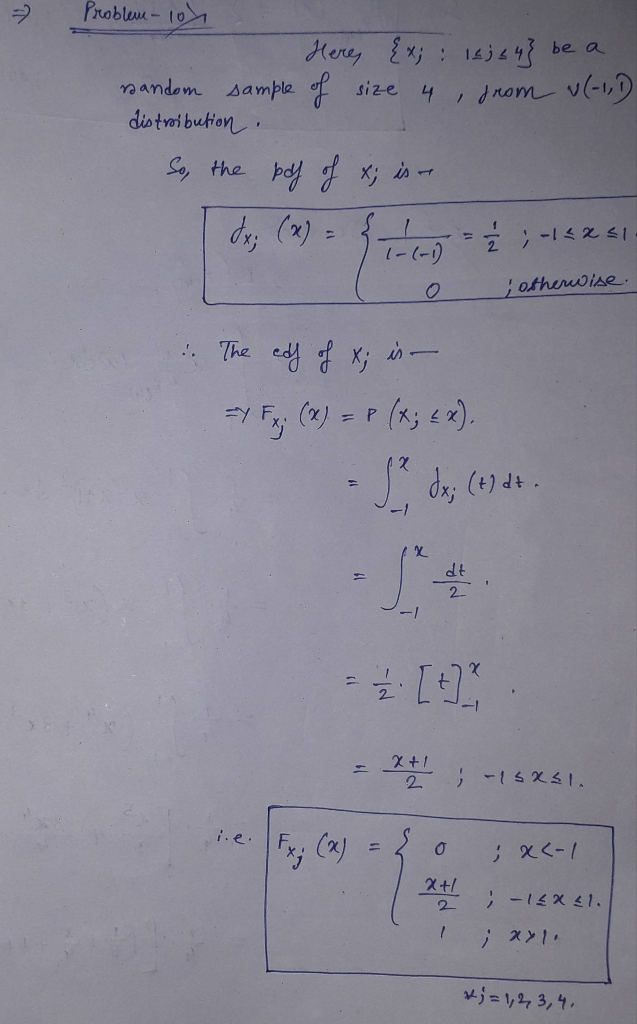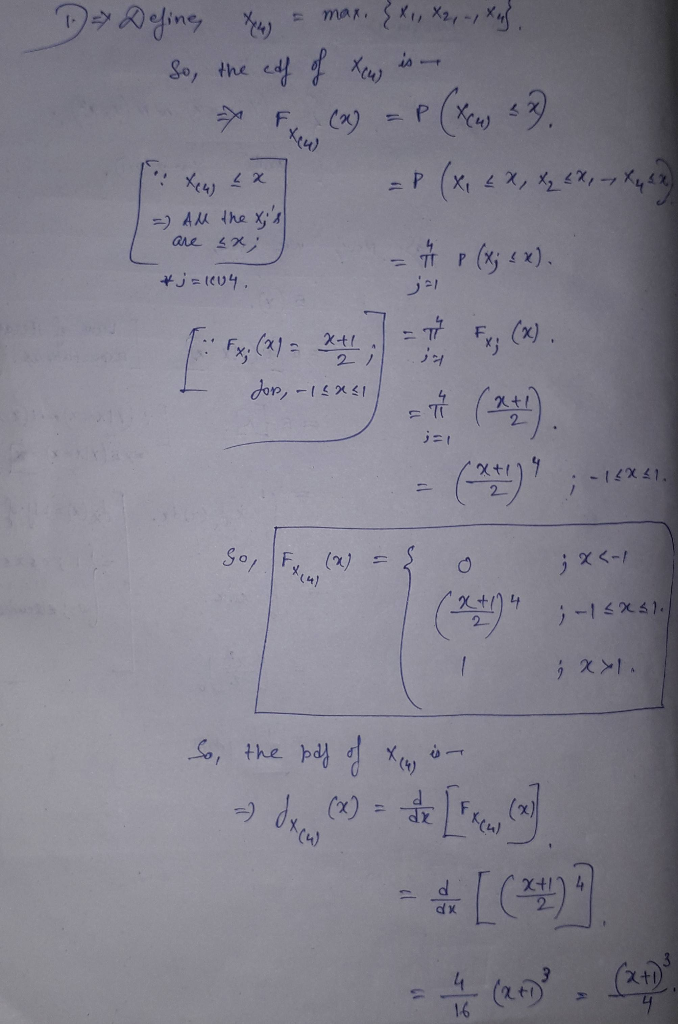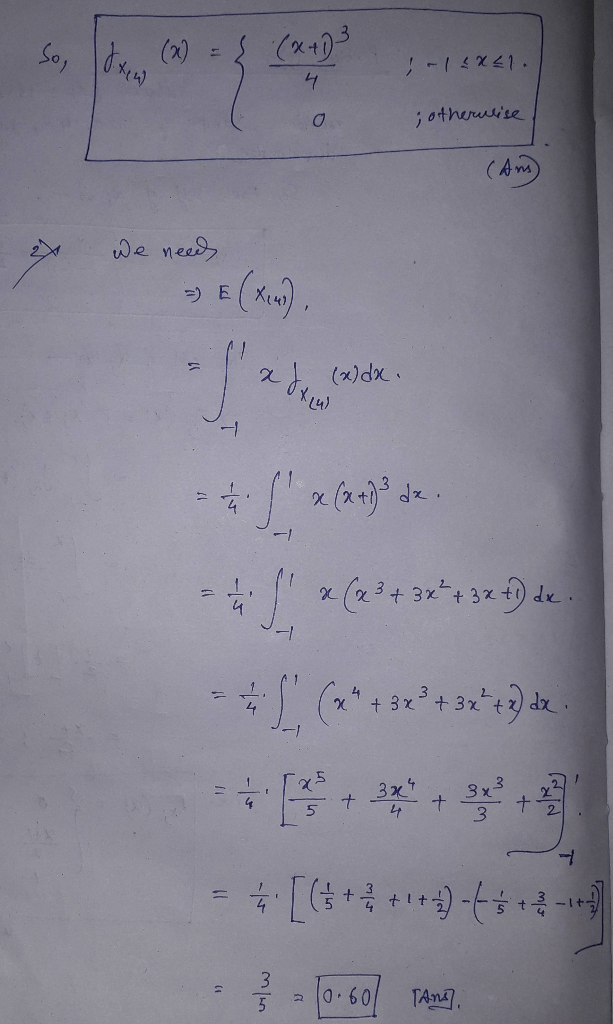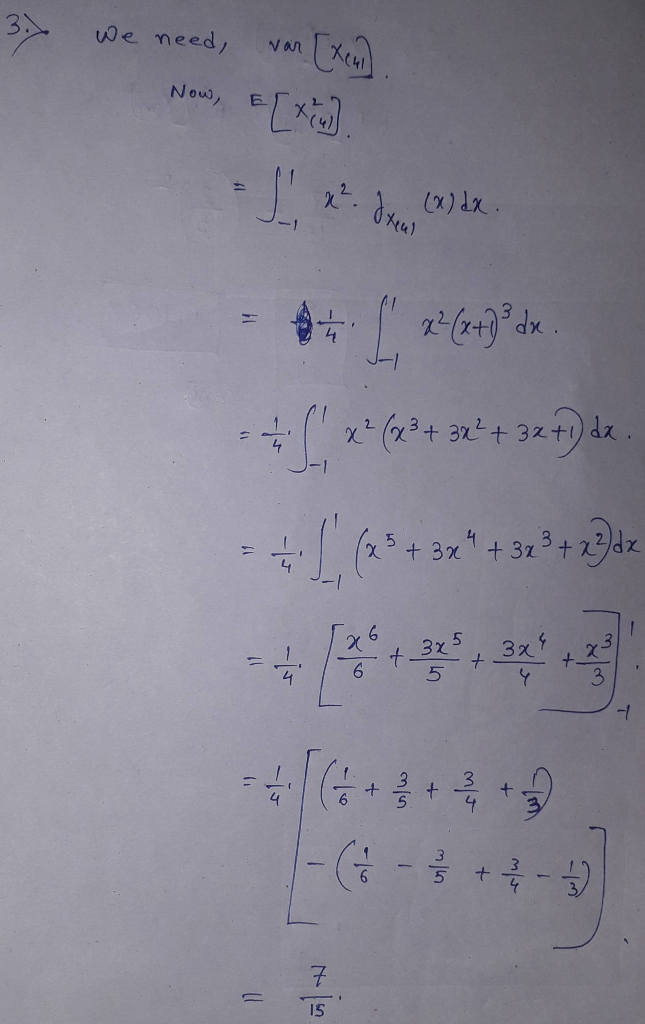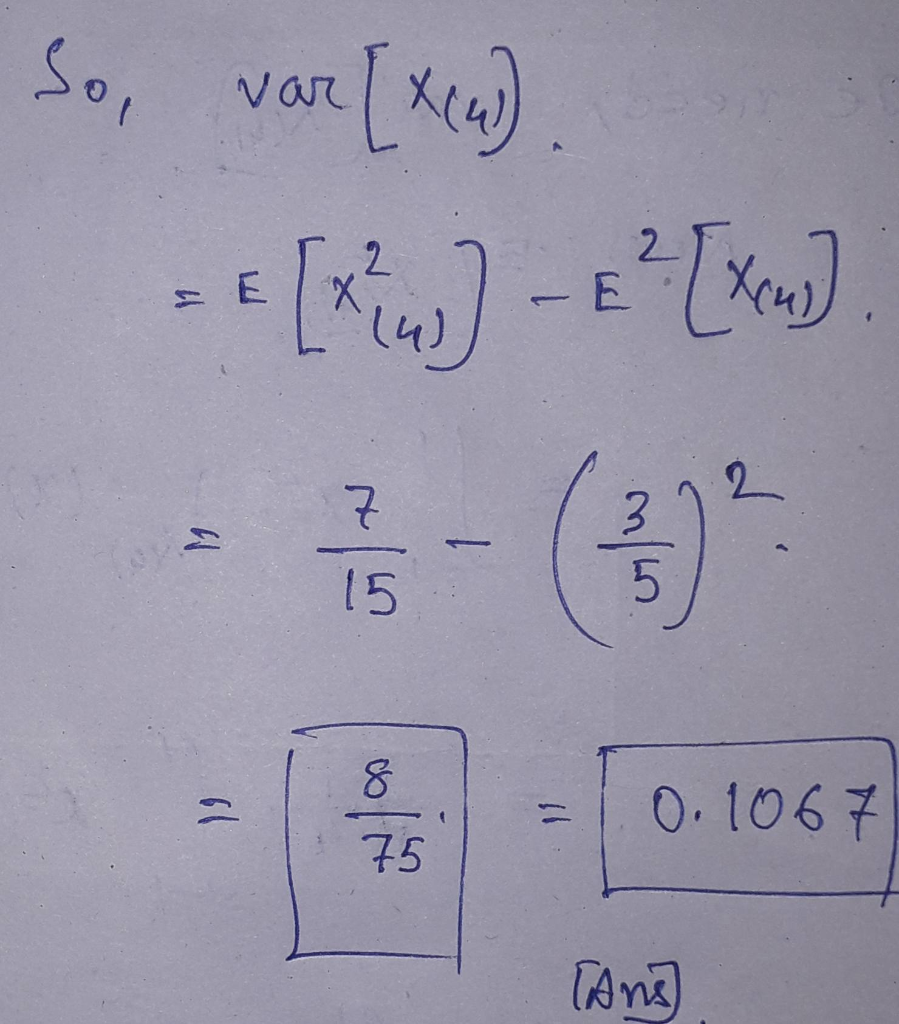##### Add Answer to: Problem 10: 10 points Assume that a sample {X;:15; <4} of size 4 is drawn from...
Similar Homework Help Questions
• ### 10. A random sample of size n = 15 is drawn from EXP(O). Find c so...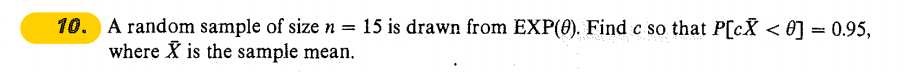10. A random sample of size n = 15 is drawn from EXP(O). Find c so that P[c# < 0) = 0.95, where X is the sample mean.

• ### [PLEASE USE HINT] Problem 4: 10 points Assume that a continuous random variable, Q, follows the...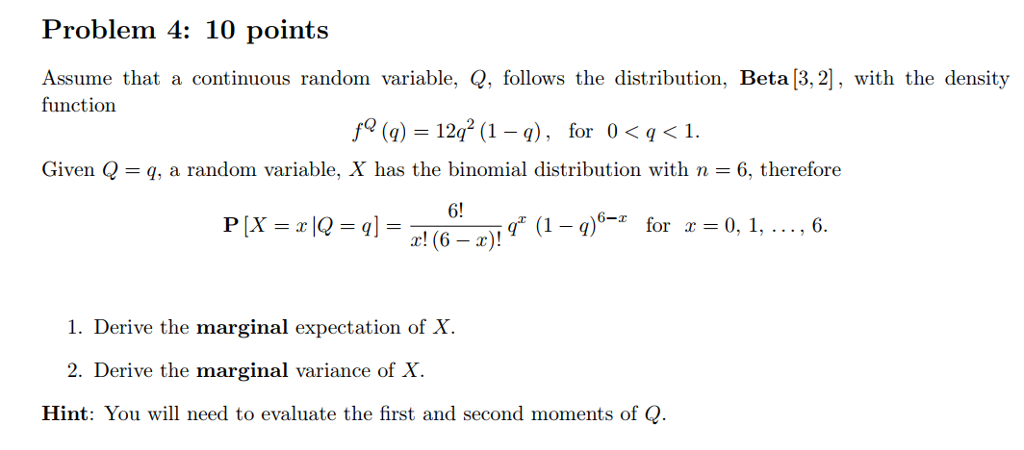[PLEASE USE HINT] Problem 4: 10 points Assume that a continuous random variable, Q, follows the distribution, Beta [3,2], with the density function /9 (q) = 12q2 (1-1), Given Q = q, a random variable, X has the binomial distribution with n = 6, therefore for 0 < q < 1. 6! r! (6-2). g" (1-q)"-z for x 0, i, . . . , 6. 1. Derive the marginal expectation of X. 2. Derive the marginal variance of X Hint:...

• ### Problem 1. 15 points] Let X be a uniform random variable in the interval [-1,2]. Let...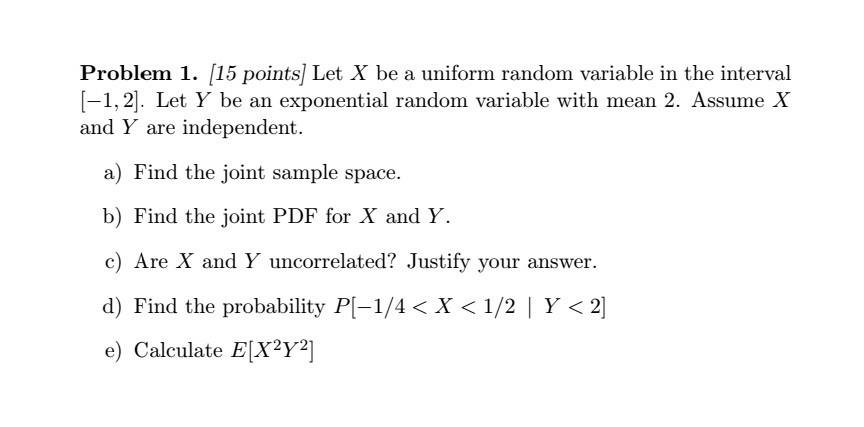Problem 1. 15 points] Let X be a uniform random variable in the interval [-1,2]. Let Y be an exponential random variable with mean 2. Assunne X and Y are independent. a) Find the joint sample space. b) Find the joint PDF for X and Y. c) Are X and Y uncorrelated? Justify your answer. d) Find the probability P1-1/4 < X < 1/2 1 Y < 21 e) Calculate E[X2Y2]

• ### 7. (1 point) Let X be the mean of a random sample of size 36 from...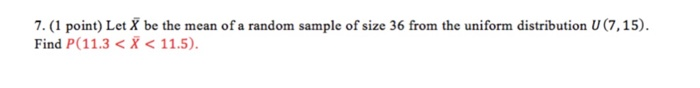7. (1 point) Let X be the mean of a random sample of size 36 from the uniform distribution U(7,15) Find P(11.3 <X < 11.5)

• ### 3. (10 points) Based on a random sample of size n from the pdf below, derive...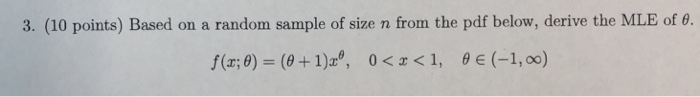3. (10 points) Based on a random sample of size n from the pdf below, derive the MLE of θ. f(z; θ)-(9 + 1)29, 0 < x < 1, θ E -1, oo)

• ### 1. (50 points) Suppose X1, ..., Xn form a random sample from a N(u,02) distribution with...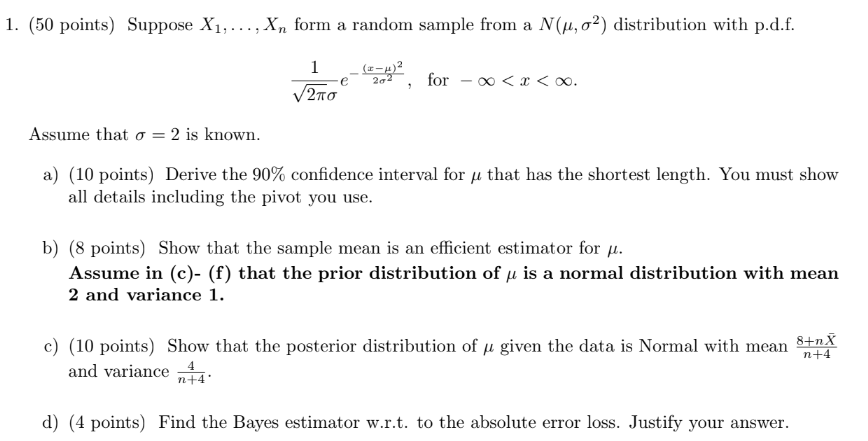1. (50 points) Suppose X1, ..., Xn form a random sample from a N(u,02) distribution with p.d.f. Fe 202, for – V2110 <x< . Assume that o = 2 is known. a) (10 points) Derive the 90% confidence interval for u that has the shortest length. You must show all details including the pivot you use. b) (8 points) Show that the sample mean is an efficient estimator for u. Assume in (c)- (f) that the prior distribution of u...

• ### Ho: u= 6 HA: u>6 2.  An observation X is drawn from a Poisson distribution...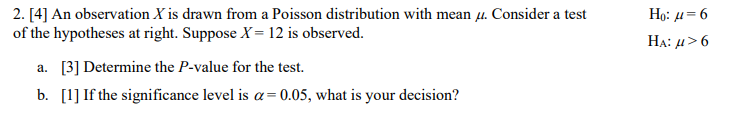Ho: u= 6 HA: u>6 2.  An observation X is drawn from a Poisson distribution with mean u. Consider a test of the hypotheses at right. Suppose X = 12 is observed. a.  Determine the P-value for the test. b.  If the significance level is a= 0.05, what is your decision?

• ### 1. Consider a random sample of size n from a population with pdf: f (x) =...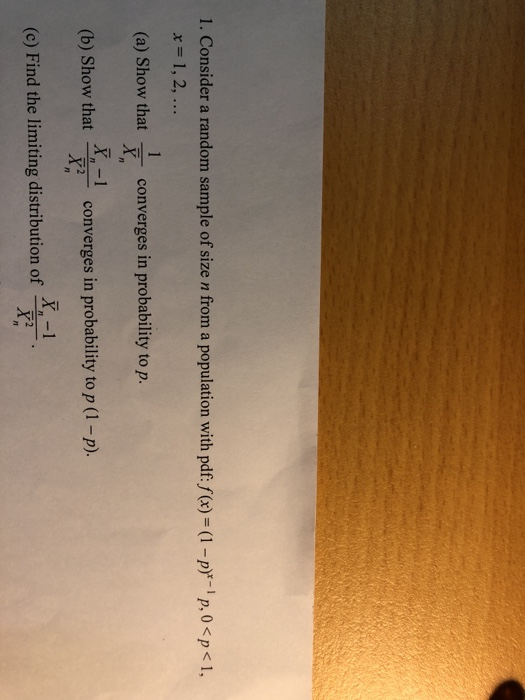1. Consider a random sample of size n from a population with pdf: f (x) = (1 -p-p, 0 <p<1, x= 1, 2, ... (a) Show that converges in probability to p. (b) Show that converges in probability to p (1 – p). (c) Find the limiting distribution of

• ### 3. (16 points) Suppose that X and Y have the following joint p.d.f. f(x,y) = for...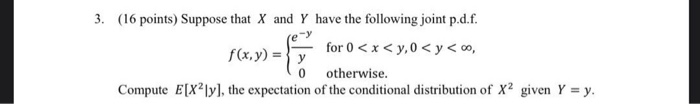3. (16 points) Suppose that X and Y have the following joint p.d.f. f(x,y) = for 0 < x < y,0 < y <, y 0 otherwise. Compute E[X2]y], the expectation of the conditional distribution of x2 given Y = y.

• ### Problem 7: 10 points Assume that the inter-arrival times, S the renewal process, j21, are independent...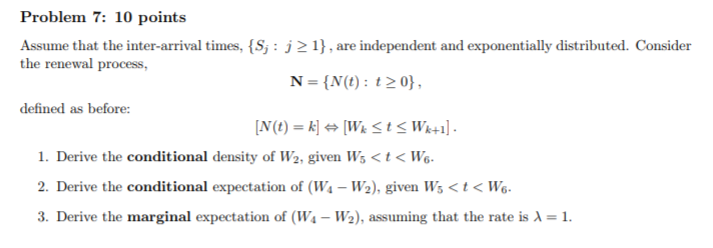Problem 7: 10 points Assume that the inter-arrival times, S the renewal process, j21, are independent and exponentially distributed. Consider N = {N(t): t 0), defined as before: 1. Derive the conditional density of W2, given Ws<t< Wo 2. Derive the conditional expectation of (Ws - W2), given Ws<t< Wo 3. Derive the marginal expectation of (W1-W2), assuming that the rate is

Free Homework App